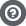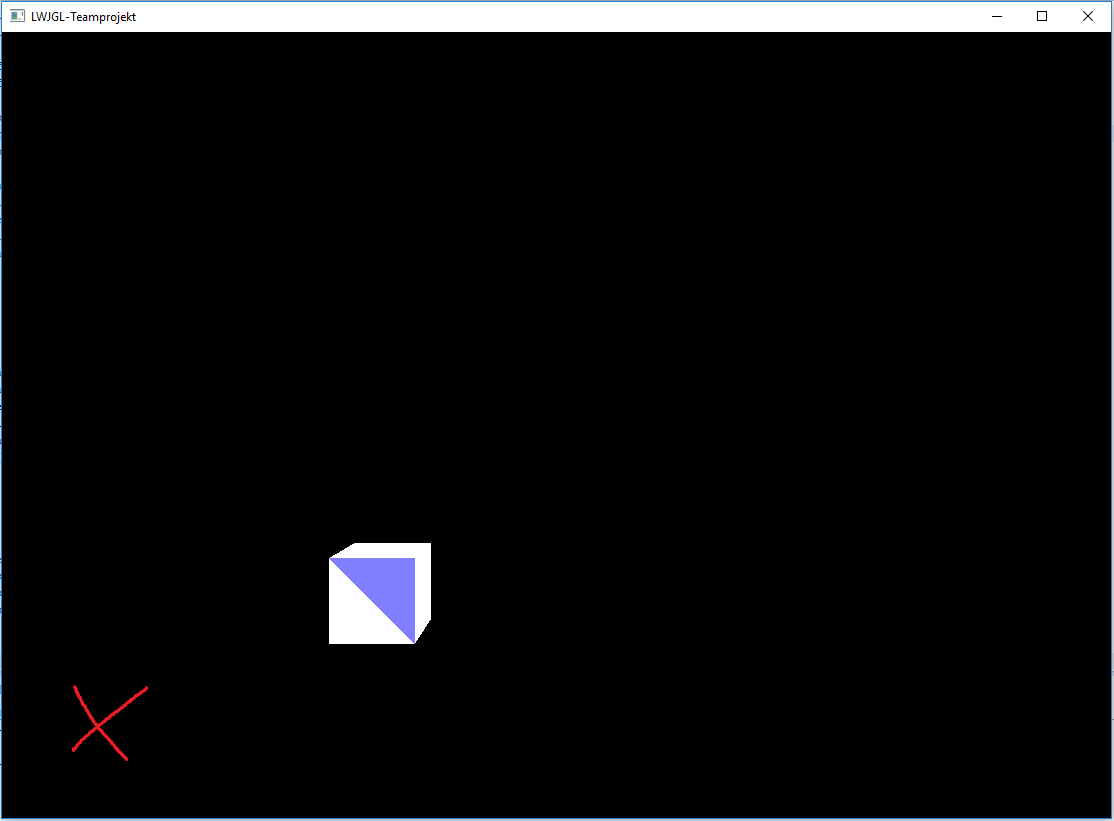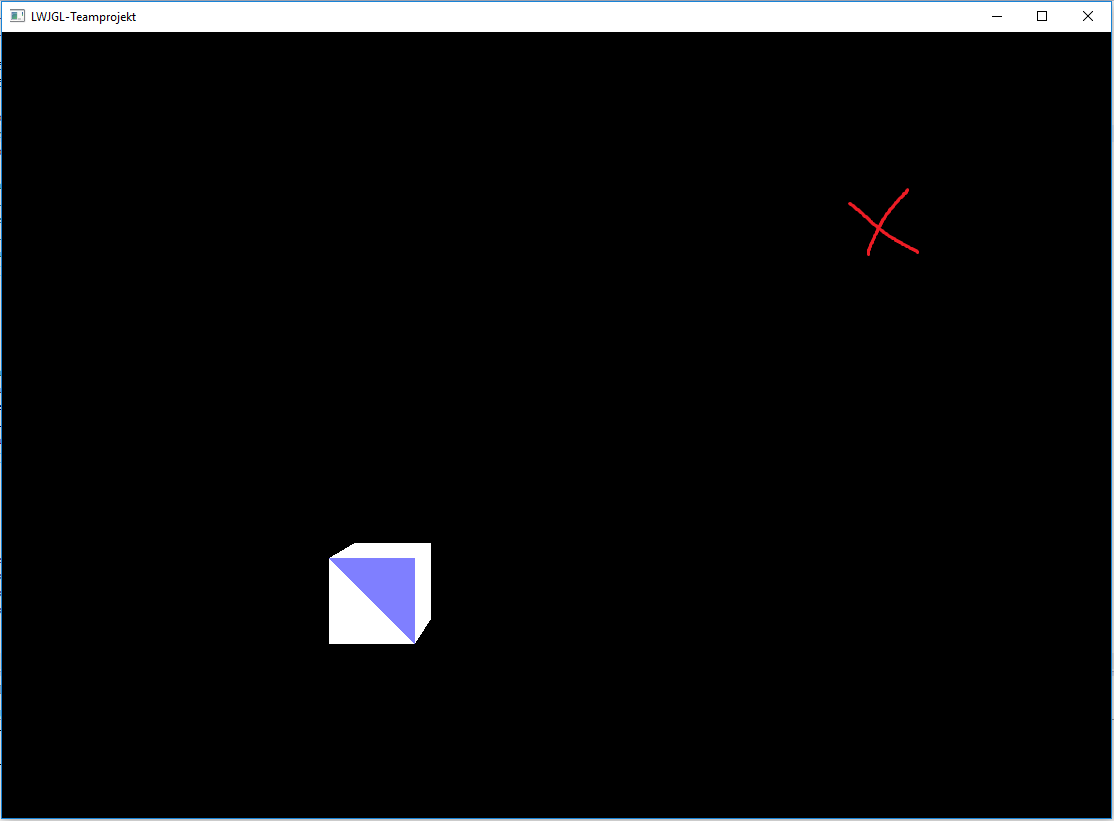Hello Guest

# Cursor Coordinates different from Object Coordinates

• 6 Replies
• 4649 Views####expensne

•• 4##### Cursor Coordinates different from Object Coordinates
« on: June 02, 2016, 10:45:14 »
Hey,

I'm pretty new to OpenGL/LWJGL and working on a little project but I'm stuck at the moment.
The goal is to load STL-Files, show them on the screen and be able to click different triangles/surfaces.

My problem now is that I rendered the object multiplied with a projection-matrix, and a transformation-matrix to move it around. These multiplications are done in the vertex-shader, is there any way to access these new coordinates of my object? Or to get coordinates of that object where the top-left corner is the (0,0) coordinate. Because the cursor position starts also at the top-left, I simply want to check if the cursos position is in between some surface coordinates.####Cornix

•• 488##### Re: Cursor Coordinates different from Object Coordinates
« Reply #1 on: June 02, 2016, 11:09:44 »
What you need is ray tracing. This is a technique where you shoot an imaginary ray from your mouse cursor through the display and then you calculate the 3d polygons it intersects with to find out which one the mouse is over.##### Re: Cursor Coordinates different from Object Coordinates
« Reply #2 on: June 02, 2016, 12:08:41 »
One way is to "unproject" the mouse cursor position (which is in OS window coordinates) to obtain a ray in model coordinates and then naively testing all model triangles against that ray.
Using JOML this then becomes:
Code: [Select]
`// Build the mvp matrix (this is your combined projection * view * model matrix)// You can build the matrix by any means you like, but it has to be the same matrix// that you send to OpenGL when rendering the model:Matrix4f mvp = new Matrix4f()  .perspective((float)Math.toRadians(60), aspectRatio, 0.1f, 100.0f) // <- any projection you like  .lookAt(0, 0, 10, 0, 0, 0, 0, 1, 0) // <- any view transformation you like  .translate(1, 2, 3).rotateY(0.125f); // <- any model transformation you like// Describe the viewport via [x, y, width, height]:int[] viewport = {0, 0, 800, 600}; // <- or whichever viewport you have// Compute OpenGL window coordinates from mouse coordinates:float winX = mouse.x;float winY = viewport - mouse.y; // <- Window's window coordinates are upside down compared to OpenGL's window coordinates// Unproject the window coordinates to a ray in model space:Vector3f origin = new Vector3f();Vector3f dir = new Vector3f();mvp.unprojectRay(winX, winY, viewport, origin, dir);// naive ray tracing algorithm: iterate over each model triangle and find the closest hitfloat minDist = Float.POSITIVE_INFINITY;Triangle closestTriangle = null;for (Triangle t : model.triangles) {  float dist = Intersectionf.intersectRayTriangle(origin, dir, t.v0, t.v1, t.v2, 1E-6f);  if (dist > 0.0f && dist < minDist) {    minDist = dist;    closestTriangle = t;  }}if (closestTriangle != null) {  Vector3f hitPosition = new Vector3f(origin).fma(minDist, dir);  // ... do something with the hit ...}`
« Last Edit: June 03, 2016, 20:12:43 by Kai »####expensne

•• 4##### Re: Cursor Coordinates different from Object Coordinates
« Reply #3 on: June 03, 2016, 19:12:01 »
Thank's guys!
I'll try it this week and report back but as far as I can see its what I'm looking for.####expensne

•• 4##### Re: Cursor Coordinates different from Object Coordinates
« Reply #4 on: June 06, 2016, 07:34:10 »
One way is to "unproject" the mouse cursor position (which is in OS window coordinates) to obtain a ray in model coordinates and then naively testing all model triangles against that ray.
Using JOML this then becomes:
Code: [Select]
`// Build the mvp matrix (this is your combined projection * view * model matrix)// You can build the matrix by any means you like, but it has to be the same matrix// that you send to OpenGL when rendering the model:Matrix4f mvp = new Matrix4f()  .perspective((float)Math.toRadians(60), aspectRatio, 0.1f, 100.0f) // <- any projection you like  .lookAt(0, 0, 10, 0, 0, 0, 0, 1, 0) // <- any view transformation you like  .translate(1, 2, 3).rotateY(0.125f); // <- any model transformation you like// Describe the viewport via [x, y, width, height]:int[] viewport = {0, 0, 800, 600}; // <- or whichever viewport you have// Compute OpenGL window coordinates from mouse coordinates:float winX = mouse.x;float winY = viewport - mouse.y; // <- Window's window coordinates are upside down compared to OpenGL's window coordinates// Unproject the window coordinates to a ray in model space:Vector3f origin = new Vector3f();Vector3f dir = new Vector3f();mvp.unprojectRay(winX, winY, viewport, origin, dir);// naive ray tracing algorithm: iterate over each model triangle and find the closest hitfloat minDist = Float.POSITIVE_INFINITY;Triangle closestTriangle = null;for (Triangle t : model.triangles) {  float dist = Intersectionf.intersectRayTriangle(origin, dir, t.v0, t.v1, t.v2, 1E-6f);  if (dist > 0.0f && dist < minDist) {    minDist = dist;    closestTriangle = t;  }}if (closestTriangle != null) {  Vector3f hitPosition = new Vector3f(origin).fma(minDist, dir);  // ... do something with the hit ...}`

It works but not like it should, I got the following problem:

I attached two images - the red X markes the spot where I clicked the mouse - as you can see always the right upper triangle gets colored. I found out that its not the mouse position that determinates which triangle gets picked, it's the middle of the screen that determinates it. On the image you can see that the middle of the screen is top right of the cube, so the right upper triangle gets colored.Here is the code I used:

Code: [Select]
` Matrix4f projDisp = renderer.getProjDispMatrix(); // the projection + transformation matrix OpenGL uses int[] viewport = {0,0, window.getWidth(), window.getHeight()}; float winX = posx; // posx <- mouse x float winY = viewport - posy; // posy <- mouse y Vector3f origin = new Vector3f(); Vector3f dir = new Vector3f(); projDisp.unproject(winX, winY, -1f, viewport, dir); // there was no unproject method without an Z coordinate so I used -1 because my Z goes into negative if I go into the room projDisp.origin(origin); // also there was no unproject method with an origin output so I used this one float minDist = Float.NEGATIVE_INFINITY; // opposite of yours because negative goes into the room Triangle closestTriangle = null; for (Triangle t : triangles) { float dist = Intersectionf.intersectRayTriangle(origin, dir, t.getV0(), t.getV1(), t.getV2(), 1E-6f); if (dist > 0.0f && dist > minDist) { minDist = dist; closestTriangle = t; } }`
« Last Edit: June 06, 2016, 07:50:13 by expensne »##### Re: Cursor Coordinates different from Object Coordinates
« Reply #5 on: June 06, 2016, 07:55:04 »
Please use Matrix4f.unprojectRay() provided by JOML 1.8.0 or 1.8.1-SNAPSHOT.
Using Matrix4f.unproject() with .origin() will give you wrong results, since the origin in post-perspective space is not the camera's position anymore.
Also, the 'minDist' has to be initialized with Float.POSITIVE_INFINITY, because Intersectionf.intersectRayTriangle() will never return a negative value 't' in the ray equation r(t) = origin + t * dir. It will only return -1.0 as special value to indicate that the ray did not hit the triangle.
And larger values for 't' (towards +inf) will always mean "farther away from the camera."
« Last Edit: June 06, 2016, 10:52:41 by Kai »####expensne

•• 4##### Re: Cursor Coordinates different from Object Coordinates
« Reply #6 on: June 08, 2016, 06:09:47 »
Please use Matrix4f.unprojectRay() provided by JOML 1.8.0 or 1.8.1-SNAPSHOT.
Using Matrix4f.unproject() with .origin() will give you wrong results, since the origin in post-perspective space is not the camera's position anymore.
Also, the 'minDist' has to be initialized with Float.POSITIVE_INFINITY, because Intersectionf.intersectRayTriangle() will never return a negative value 't' in the ray equation r(t) = origin + t * dir. It will only return -1.0 as special value to indicate that the ray did not hit the triangle.
And larger values for 't' (towards +inf) will always mean "farther away from the camera."

Oh sorry didn't realized that. Thanks so mutch it works perfect!